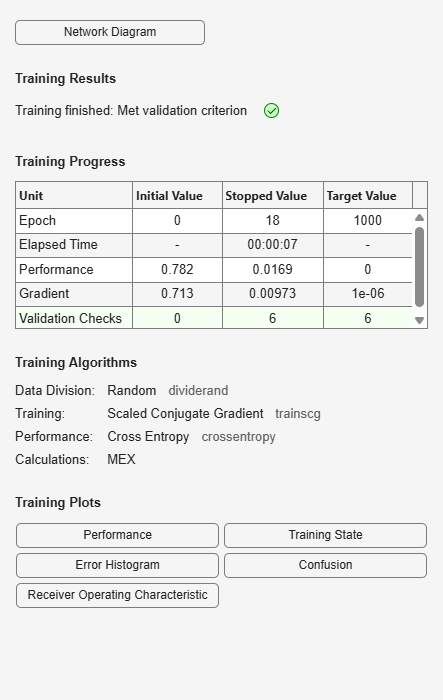# plotroc

## Syntax

``plotroc(targets,outputs)``
``plotroc(targets1,outputs2,'name1',...)``

## Description

example

````plotroc(targets,outputs)` plots the receiver operating characteristic for each output class. The more each curve hugs the left and top edges of the plot, the better the classification.```
````plotroc(targets1,outputs2,'name1',...)` generates multiple plots.```

## Examples

collapse all

This example shows how to plot the receiver operating characteristic for each output class in a pattern network.

```load simplecluster_dataset net = patternnet(20); net = train(net,simpleclusterInputs,simpleclusterTargets); simpleclusterOutputs = sim(net,simpleclusterInputs); plotroc(simpleclusterTargets,simpleclusterOutputs)```## Input Arguments

collapse all

Network targets, specified as a matrix or cell array.

Network outputs, specified as a matrix or cell array.